# Energy Stored in Inductor – How Does Inductor Store Energy?

Posted on

How energy stored in inductor? An inductor is also named as a reactor, coil and choke. It is a two terminal electrical component used in various electrical and electronic circuits. Usually, energy stored in inductor in the form of a magnetic field. It comprises of a wire, usually twisted into a coil. When a current passes through it, energy stored temporarily in the coil.

An inductor is a passive electronic component which is capable of storing electrical energy in the form of magnetic energy. Basically, it uses a conductor that is wound into a coil, and when electricity flows into the coil from the left to the right, this will generate a magnetic field in the clockwise direction.

# Energy Stored in Inductor – How Does Inductor Store Energy?

Inductor is basically a coil of wire. It hates change in current passing through it , so if current tries to change then inductor corrects it by generating an induced current in the coil in the direction of current (so that they add up) if current is dropping and in opposite direction to the current if current is increasing (so that it may cancel the extra current).

## How Does Inductor Store Energy?

This happens as follows:

Assume we have a inductor connected to an ac source. Lets say a sinewave.

Then, as the current starts to increase then magnetic fields inside inductor also increase this change in magnetic field generates some induced current in the opposite direction of main current so as to cancel the effect of increasing current.

When the current starts to drop in next half cycle the decreasing magnetic fields induce current in the same direction of main current.

Therefore basically inductor is trying to minimize the changes in the current by storing energy when current is in excess (reducing current to normal value) and supplyin it in the form of current when main current is decreasing (increasing the net current to normal value).

## Energy Stored in Inductor in Electric Circuits

Both the capacitor and inductor are basic elements of electric circuits. Both are reactive elements in the sense that in the capacitor i=C dv/dt while in the inductor v=-L di/dt. Notice the role of v and i are reversed in the the two components.

This is because the physical nature of both elements where the capacitor reacts its changes by developing electrostatic field the inductor reacts by changing its magnetic field.

The electrostatic field is created by the electric charges stored in the  capacitor. NOT only electrons are stored but also an equal positive charges must be also stored in it. The magnetic field in the inductor is created by the current flowing in it. The electric field stores energy and also does the magnetic field as formulated above in the question.

Consequently, it is very important  to notice that the energy is stored in the capacitor  as a potential energy while the energy stored in the inductor is kinetic energy.

The analogy to the mechanical system the capacitor  is analog to the spring and the inductor to the flywheel or in general to a moving mass. The L-C circuit is similar to the sprig mass oscillator system.

## Energy Stored in Inductor Formula

Suppose that an inductor of inductance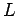is connected to a variable DC voltage supply. The supply is adjusted so as to increase the currentflowing through the inductor from zero to some final value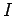.

As the current through the inductor is ramped up, an emf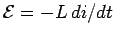is generated, which acts to oppose the increase in the current. Clearly, work must be done against this emf by the voltage source in order to establish the current in the inductor. The work done by the voltage source during a time intervalis: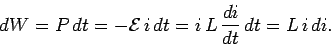Here,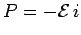is the instantaneous rate at which the voltage source performs work. To find the total work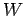done in establishing the final currentin the inductor, we must integrate the above expression. Thus: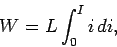giving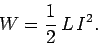This energy is actually stored in the magnetic field generated by the current flowing through the inductor. In a pure inductor, the energy is stored without loss, and is returned to the rest of the circuit when the current through the inductor is ramped down, and its associated magnetic field collapses.

Consider a simple solenoid. Can be combined to give:Which reduces to: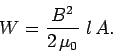It turns out that this result is quite general. Thus, we can calculate the energy content of any magnetic field by dividing space into little cubes (in each of which the magnetic field is approximately uniform), applying the above formula to find the energy content of each cube, and summing the energies thus obtained to find the total energy.

When electric and magnetic fields exist together in space. Can be combined to give an expression for the total energy stored in the combined fields per unit volume:### Energy Stored in Inductor Conclusion

After going through the above portion of energy stored in inductor we can now establish how inductor store energy. I hope you enjoy when reading this article, thank you.